### Transient Vibrations: Response of Spring–Mass System to an Exponential Decay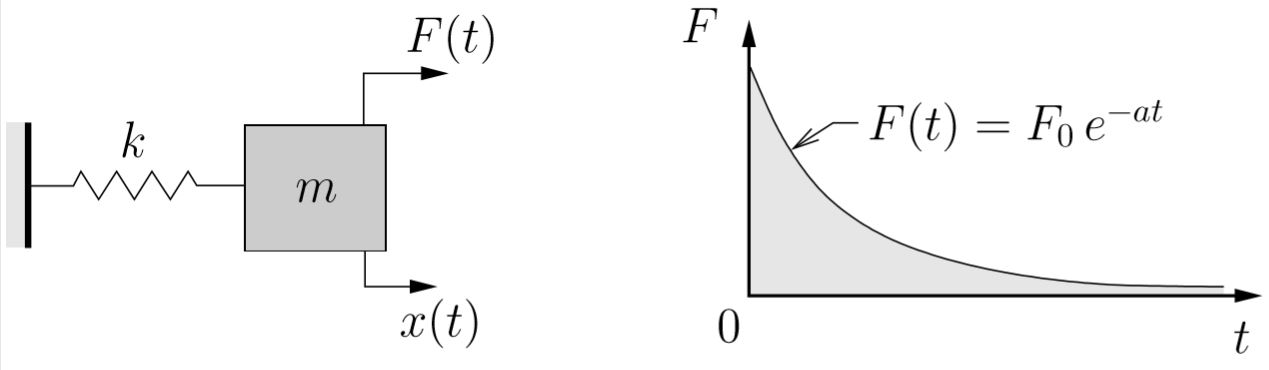Exponential Decay

The equation of motion in this case is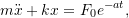for which the particular solution can be shown to be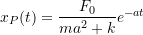so that the total solution becomes

(7.7)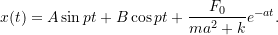If the initial conditions are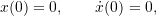then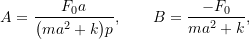and we get

(7.8)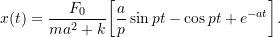or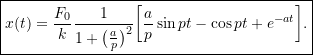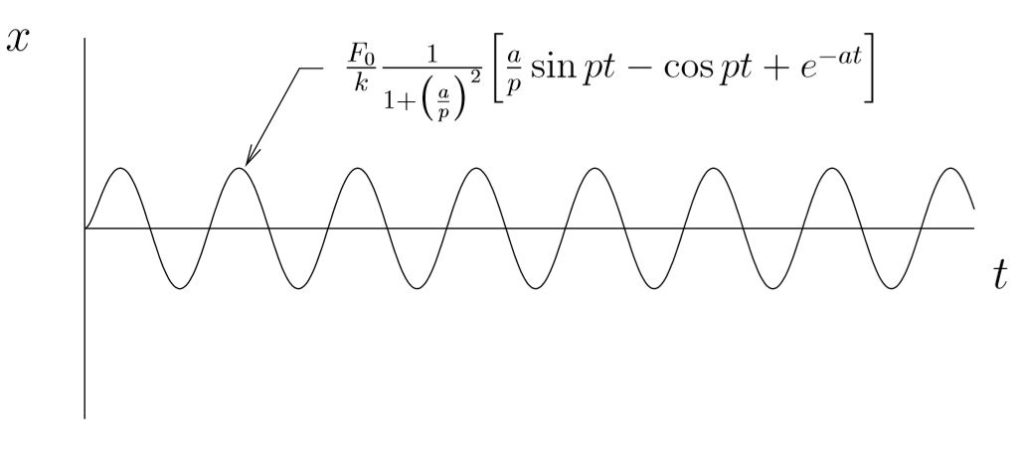Response to Exponential Decay Function with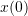=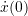= 0